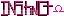-=+=- -=+=- -=+=- -=+=- -=+=- -=+=- -=+=- -=+=- -=+=- -=+=- -=+=- -=+=- -=+=- -=+=- -=+=- -=+=- -=+=- -=+=- -=+=- -=+=- -=+=- -=+=- -=+=- -=+=- -=+=- -=+=- -=+=- -=+=- -=+=- -=+=- (c) WidthPadding Industries 1987 0|617|0 -=+=- -=+=- -=+=- -=+=- -=+=- -=+=- -=+=- -=+=- -=+=- -=+=- -=+=- -=+=- -=+=- -=+=- -=+=- -=+=- -=+=- -=+=- -=+=- -=+=- -=+=- -=+=- -=+=- -=+=- -=+=- -=+=- -=+=- -=+=- -=+=- -=+=-
SoCoder -> Showcase Home -> Graphic Demos

StealthCreated : 21 November 2006
Edited : 21 November 2006
System : Windows
Language : Blitz

### Visualizer Demo

Visualizer Demo
ScreenshotsThis is a music visualizer that I have been working on for a music player. Its not the graphical aspect that makes this cool (because it lacks that). Its the ability to "see" your music play. It is very calm when the music is calm. Theres lots of chaos when the music is fast. It also switches between two colors when the depending if the song is calm or noisy. Something else that I think is impressive, is it does everything in real time. Let me tell you, there are a lot of crazy math formulas going in to this program. Each pixel requires 5 math equations to blur, per frame. Thats about 6,000,000 calculations a second (well if it runs at 30fps). Lots and lots of thinking went in to this visualizer... Tell me what you think after you watch it.To move the window, click down on it and drag it.

(You need your own MP3 files. You will be prompted to open one when you run the program.)

Tuesday, 21 November 2006, 18:47
JayenkaiWorks great with War of the WorldsWednesday, 22 November 2006, 13:24
SchererererThat's pretty cool! It's worthy of a WMP or Winamp visualization. Looks very good playing Sandstorm imhoWednesday, 22 November 2006, 14:15
StealthThanks for the feedback!Wednesday, 22 November 2006, 15:24
moleI think its wicked, although as i said before, it finds an Enya song more frantic than a metallica song (yes, a pretty heavy metallica song) but meh, its all prettyWednesday, 22 November 2006, 16:20
Stealth@mole

It follows more of the beat and less of the loudness of the song. I don't know if that helps explain why.Wednesday, 22 November 2006, 23:33
magicmanwow, comming along great!